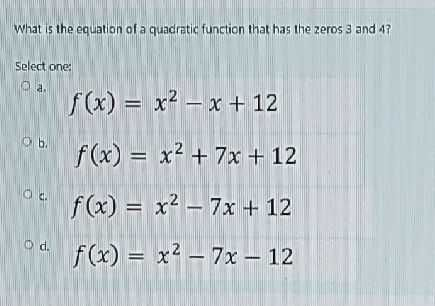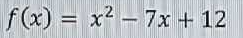### Still have math questions?

Algebra
QuestionWhat is the equation of a quadratic function that has the zeros $$3$$ and $$4$$ ?

Select one:

a. $$f ( x ) = x ^ { 2 } - x + 12$$

b. $$f ( x ) = x ^ { 2 } + 7 x + 12$$

c. $$f ( x ) = x ^ { 2 } - 7 x + 12$$

d. $$f ( x ) = x ^ { 2 } - 7 x - 12$$